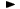ADVFN HomeHelpFinancialsDeeper AnalysisFINANCIAL RATIOS - Liquidity RatiosCurrent Ratio

# Current Ratio

Current ratio, expresses a company's ability to repay short-term creditors out of its total current assets. The current ratio is the result of dividing the total of current assets (incl. stocks) by short-term borrowings. It shows the number of times short-term liabilities are covered by current assets. If the value is greater than 1.00, it means fully covered.

The formula is as follows:

= (stocks + debtors + cash & equivalents + current assets,other) / creditors,short